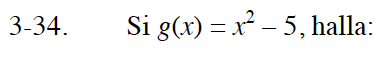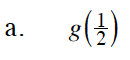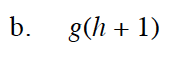### Home > INT3S > Chapter 3 > Lesson 3.1.2 > Problem3-34

3-34.
1. Si g(x) = x2 – 5, halla: Homework Help (ESP) ✎

1. g()

2. g(h + 1)$g\left(\frac{1}{2}\right)=\left(\frac{1}{2}\right)^2-5=?$g(h + 1) = (h + 1)2 − 5 = ?